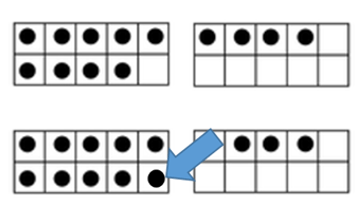## Composing & Decomposing Elementary Examples

Numbers can be composed and decomposed in different ways.

In the 5-Frame or 10-Frame model, learners can begin developing a visual benchmark for parts of numbers and how they are related. Marion Small (2010)1 suggests starting the play by putting counters anywhere on the frame. “Eventually, we lead students to fill the frame from the left. We help them to observe how this not only makes it easier to quickly recognize how many there are because the configurations become familiar,” but also to focus on relationships between numbers.“Seven is two more than five or three fewer than ten.”Use counters to represent addends (e.g. 9+4). Move counters from one frame to another to make ten. Then add: 10+3.

When learners become flexible in knowing that numbers can be visualized in parts, this strategy can be applied to basic fact understanding (versus memorization), toward operating mentally with larger numbers.2

For instance, 32 – 6. You could decompose 6 into 2 and 4. 32 – 2 = 30.  30 – 4 = 26.

Or, 23 – 19. You could add instead. 19+1=20, plus 3 more to 23. 19 + 4 = 23, so 23 – 19 = 4. (Humphreys & Parker, 2015)Shapes can be decomposed, as well. Consider the net of a rectangular prism that includes rectangles and squares.